# 如何理解堆

• 必须是一棵完全二叉树，除了最后一层，其他节点的个数都是满的，最后一层的节点都靠左排列
• 树种的每一个节点都必须大于等于或者小于等于其子树的每个节点的值，对于每个节点的值大于等于子树每个节点值得堆叫做大顶堆，小于等于书中每个节点的值叫做小顶堆

# 如何实现一个堆

## 操作

### 往堆中插入一个元素``````public class Heap {
private int count; // 当前堆中存储元素的个数
private int[] data;
private int capacity;

public Heap(int capacity) {
this.data = new int[capacity + 1];
this.capacity = capacity;
this.count = 0;
}

public void swap(int[] nums, int x, int y) {
int temp = nums[y];
nums[y] = nums[x];
nums[x] = temp;
}

public void insert(int value) {
if (count >= capacity) {
return;
}
count++;  // 个数加1
data[count] = value; // 堆的末尾添加新的元素
int i = count;  // 从下往上开始堆化
// i/2 表示父节点的索引，如果大于零并且子节点的值大于父节点的值进行交换
while (i / 2 > 0 && data[i] > data[i / 2]) {
swap(data, i / 2, i);
i = i / 2;
}
}
``````

### 删除堆顶元素``````public void removeMax() {
if (count == 0) {
return;
}
data = data[count];
count--;
heapify(data, count, 1);
}

private void heapify(int[] nums, int n, int i) {

while (true) {
int maxPos = i;
if (2 * i <= count && nums[i] < nums[2 * i]) { // 和左子节点进行比较
maxPos = 2 * i;
}
if (2 * i <= count && nums[i] < nums[2 * i] + 1) { // 和右子节点进行比较
maxPos = 2 * i + 1;
}
if (maxPos == i) { // 没有子节点了
break;
}
swap(nums, i, maxPos); // 交换数据
i = maxPos; // 初始化到交换的位置
}
}
``````

# 如何基于堆实现排序

## 堆排序的过成可以分为两个步骤：建堆和排序

### 建堆

``````private void heapify(int[] nums, int n, int i) {

while (true) {
int maxPos = i;
if (2 * i <= count && nums[i] < nums[2 * i]) { // 和左子节点进行比较
maxPos = 2 * i;
}
if (2 * i <= count && nums[i] < nums[2 * i] + 1) { // 和右子节点进行比较
maxPos = 2 * i + 1;
}
if (maxPos == i) { // 没有子节点了
break;
}
swap(nums, i, maxPos); // 交换数据
i = maxPos; // 初始化到交换的位置
}
}

/**
* 建堆的过程，自上而下，如果从叶子节点开始就是自己和自己比较
* 所以应该从第一个非叶子节点开始，对于完全二叉树，第一个非叶子节点的位置就是 n/2
* @param nums
* @param n
*/
public void buildHeap(int[] nums, int n){
for (int i = n / 2; i >=1 ; i--) {
heapify(nums, n , i);
}

}
``````S 的中间部分是一个等比数列，所以最后可以用等比数列的求和公式来计算，最终的结果就是下面图中画的这个样子.### 排序``````public void sort(int[] nums, int n) {
buildHeap(nums, n);
int k = n;
while (k > 1) {
swap(nums, 1, k); // 将根元素和最后一个元素进行交换
k--;
heapify(nums, k, 1); // 交换后对第一个元素进行堆化
}
}
``````

##### 热门推荐
•浏览(919)
•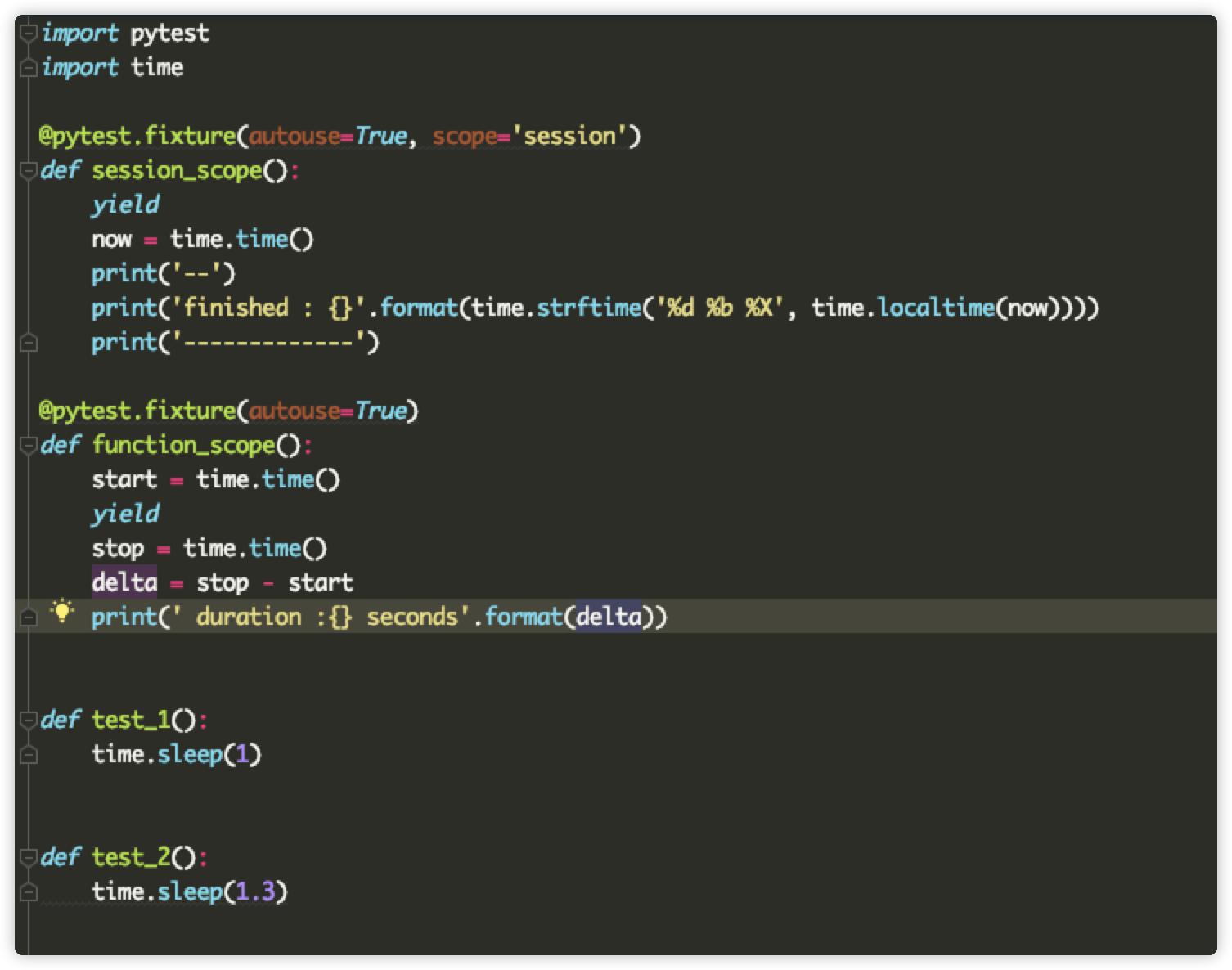浏览(521)
•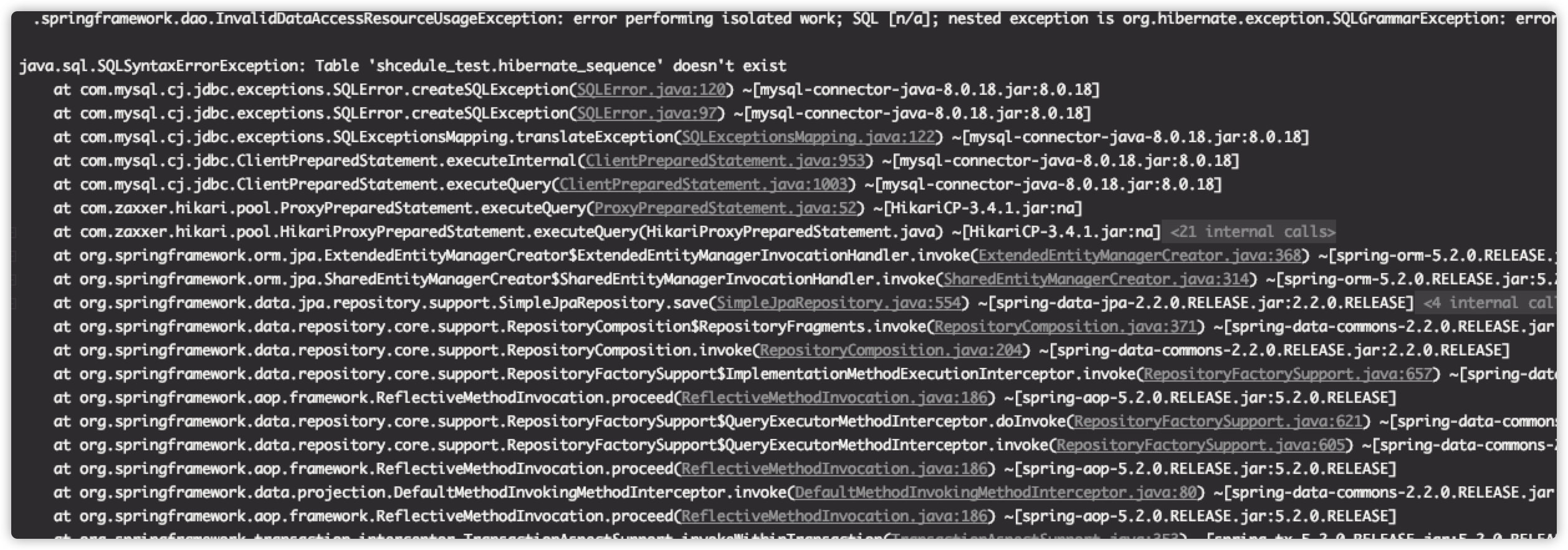浏览(482)
•浏览(450)
•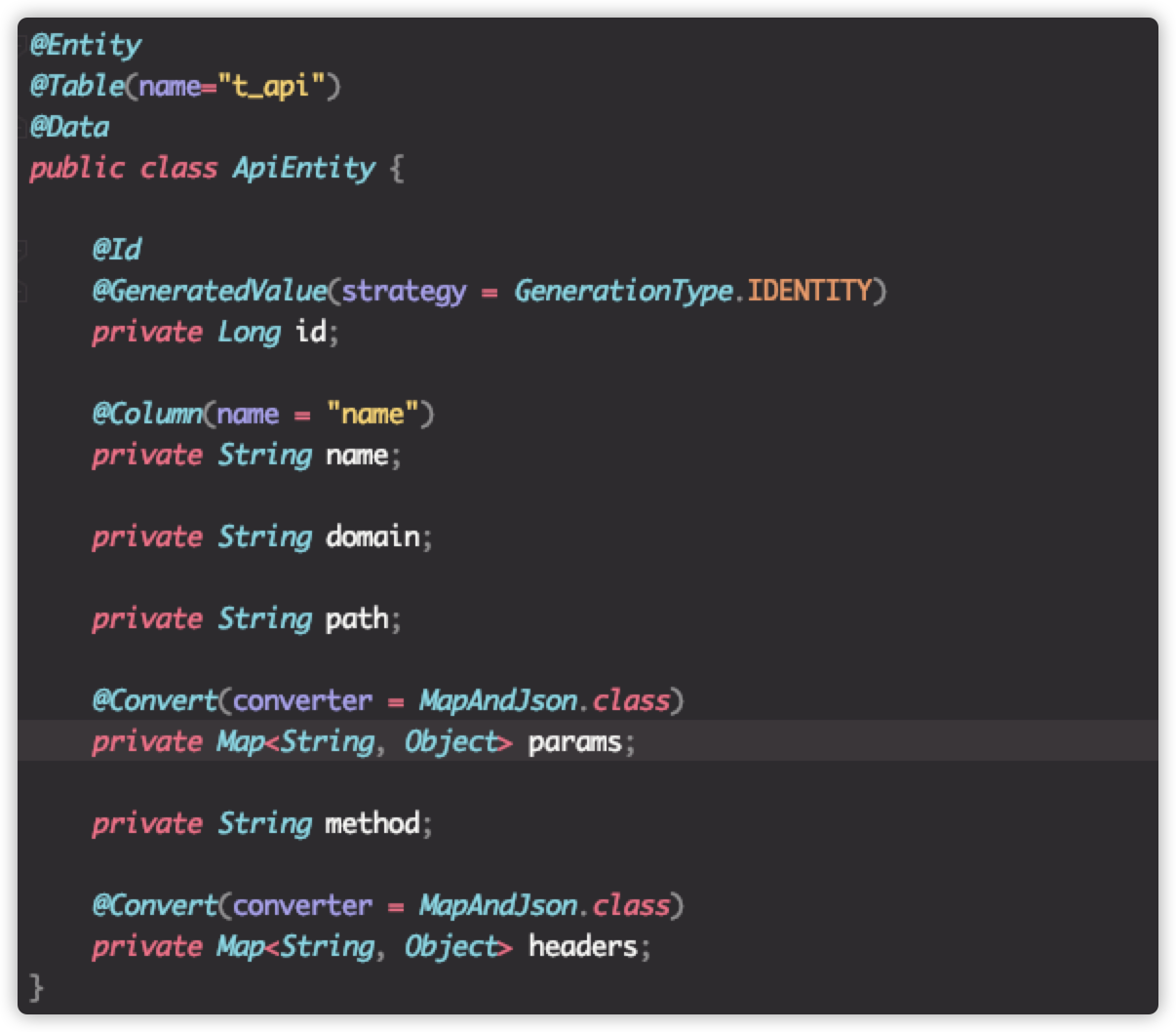浏览(393)
•浏览(381)
•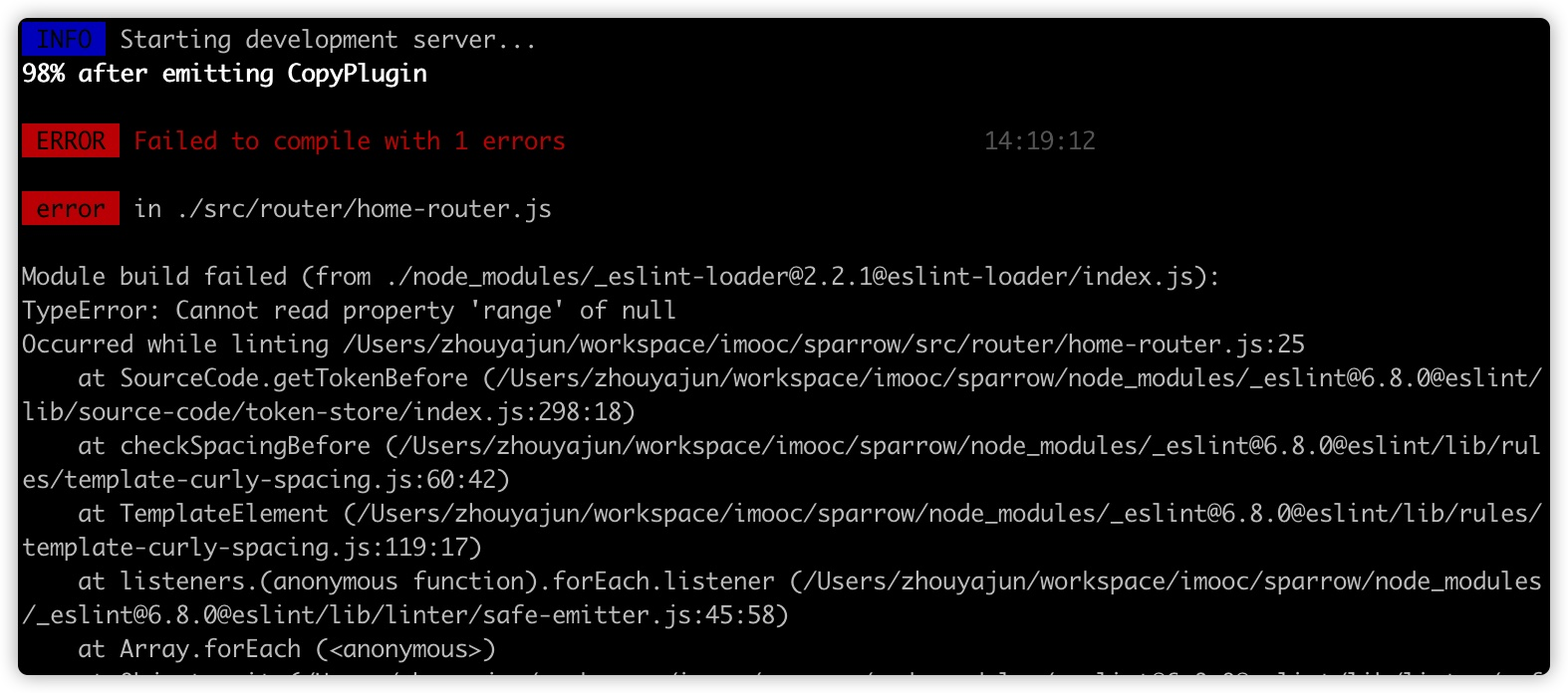浏览(316)
•浏览(287)
•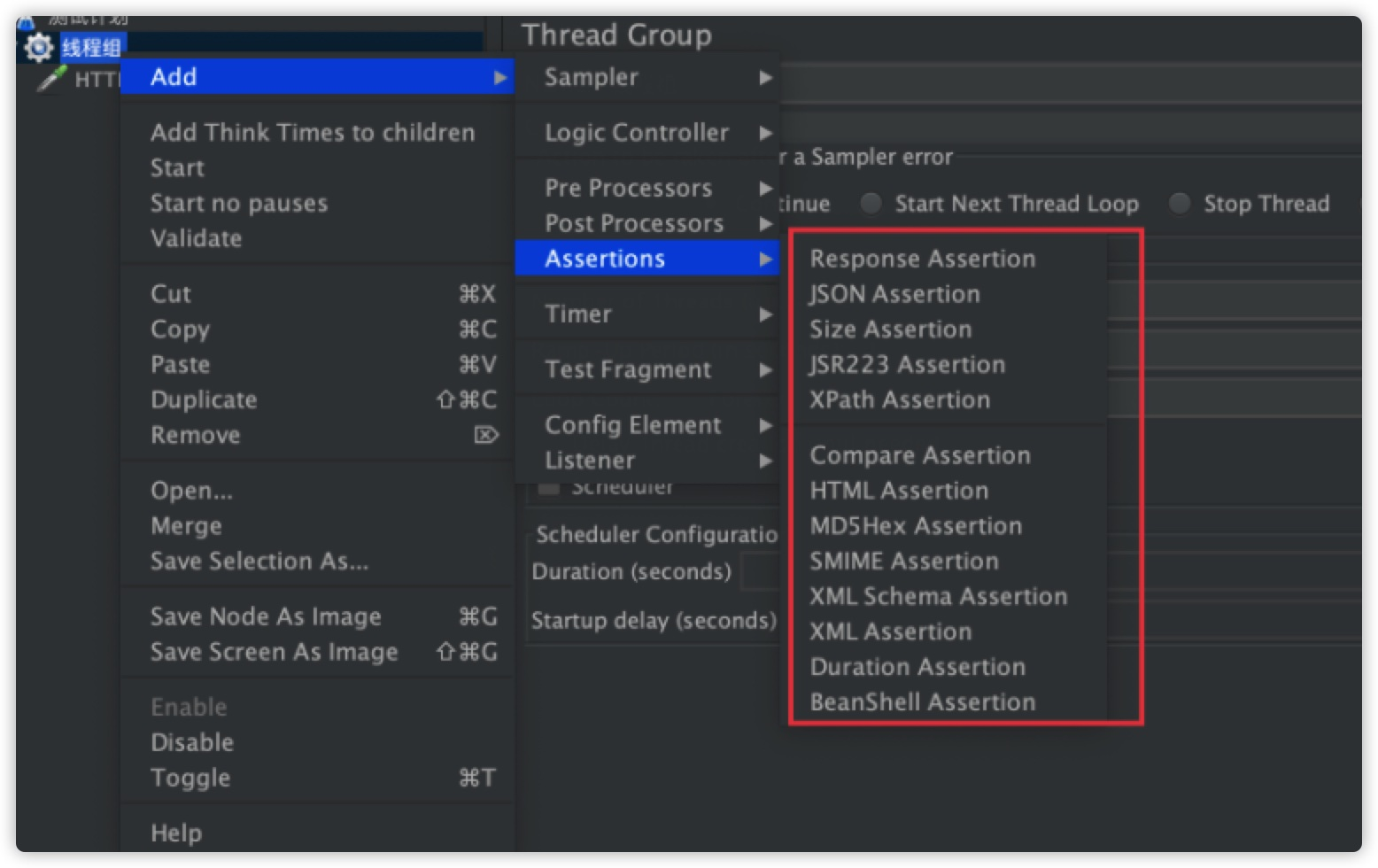浏览(235)
•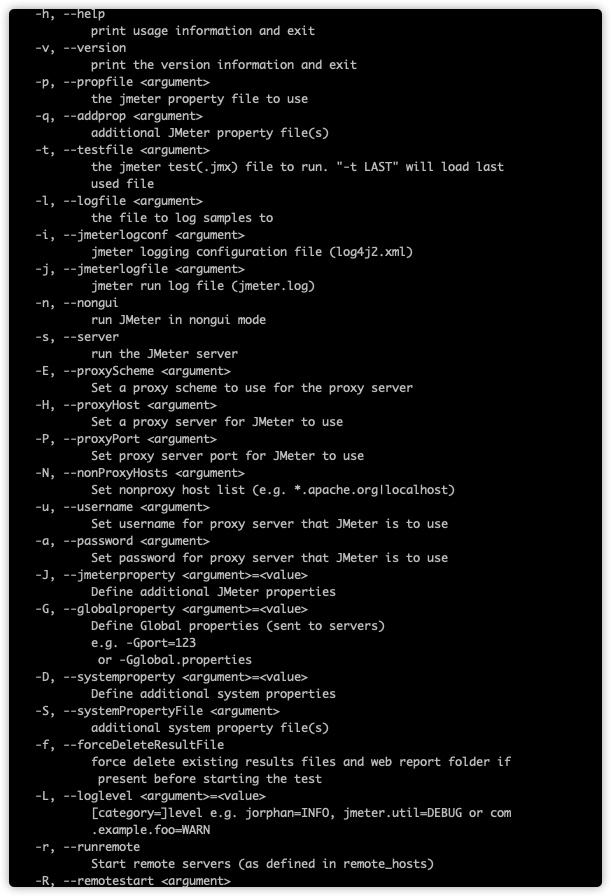浏览(234)